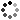# 8th Grade Math Review Activities

On Sale
\$5.00
\$5.00
Preview

Your 8th grade math students will love these solve and sort puzzles to review various 8th grade math concepts. Includes puzzles for functions, Pythagorean theorem, rational and irrational numbers, equations with variables on both sides, slope, systems of equations, and volume of cones, cylinders and spheres. These work great for stations. Perfect for the end of 8th grade math or the beginning of 9th grade math.

Includes:

• 6 puzzles which review the following:
• Functions
• Pythagorean theorem and rational/irrational numbers
• Equations with variables on both sides
• Slope
• Systems of equations
• Volume of cylinders, cones and spheres
• Each puzzle includes 16 problems

Highlights

• Helps build procedural fluency
• Perfect for stations, class work, or homework

Please feel free to contact me with any questions!

Michelle,

Make Sense of Math

One purchase is for one teacher/family. You may only use this item with students in a single classroom/family.